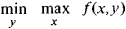# minimax

(redirected from minimaxes)
Also found in: Dictionary.
Related to minimaxes: Minimax algorithm

## minimax

[′min·ə‚maks]
(mathematics)
The minimum of a set of maxima.
In the theory of games, the smallest of a set of maximum possible losses, each representing the most unfavorable outcome of a particular strategy.

## Minimax

in mathematics, the value of the expressionof a real function f(x,y) of two variables. The concept of maximin equal to maxy minxf(x, y) is related to the concept of minimax. In the theory of zero-sum games, the fundamental optimality principle is the minimax principle, which consists in the attempt of a player to minimize his loss, assuming that his opponent’s strategy takes the most unfavorable form.

## minimax

(games)
An algorithm for choosing the next move in a two player game. A player moves so as to maximise the minimum value of his opponent's possible following moves. If it is my turn to move, I give a value to each legal move I might make. If the result of a move is an immediate win for me I give it positive infinity and, if it is an immediate win for you, negative infinity. The value to me of any other move is the minimum of the values resulting from each of your possible replies.

The above algorithm will give every move a value of positive or negative infinity since the value of every move will be the value of some final winning or losing move. This can be extended if we can supply a heuristic evaluation function which gives values to non-final game states without considering all possible following complete sequences. We can then limit the minimax algorithm to look only a certain number of moves ahead. This number is called the "look-ahead" or "ply".